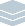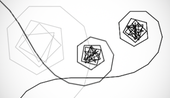Wolfram Archive
Wolfram Programming Lab is a legacy product.
All the same functionality and features, including access to Programming Lab Explorations, are available with Wolfram|One.
Start programming now. »# Tangled Turtle

Find the paths for a simulated turtle that turns more at each step.

Run the code to make a table of increasing angles. Try other step sizes:

Make a list of angles from 0 to 120 degrees in steps of 30 degrees:

Table[n Degree, {n, 0, 120, 30}]

Table[n Degree, {n, 0, 120, 30}]

Find the path taken by a series of steps at these successive angles. Try other step sizes:

This gives the sequence of points encountered by starting at {0,0} headed east, turning 0°, moving 1 unit, turning 30°, moving 1 unit, turning 60°, moving 1 unit, etc.:

AnglePath[Table[n Degree, {n, 0, 120, 30}]]

AnglePath[Table[n Degree, {n, 0, 120, 30}]]

Draw the path:

Draw a line through the points along the path. This is sometimes referred to as turtle geometry, since you can think of it as the path taken by a turtle that follows a sequence of (turn, move) instructions:

Graphics[Line[AnglePath[Table[n Degree, {n, 0, 120, 30}]]]]

Graphics[Line[AnglePath[Table[n Degree, {n, 0, 120, 30}]]]]

Use 5° increments. Try smaller and larger ones:

Using a smaller increment gives a path with more segments and whose angle changes more slowly, giving a smooth spiral at the beginning:

Graphics[Line[AnglePath[Table[n Degree, {n, 0, 120, 5}]]]]

Graphics[Line[AnglePath[Table[n Degree, {n, 0, 120, 5}]]]]

Try increasing the maximum angle. Try other maxima:

Increasing the maximum angle gives a longer path... up to a point. Eventually this path retraces itself:

Graphics[Line[AnglePath[Table[n Degree, {n, 0, 1000, 5}]]]]

Graphics[Line[AnglePath[Table[n Degree, {n, 0, 1000, 5}]]]]

Make an interactive version. Drag the sliders to change the path shape:

This is the code to draw a path up to a maximum angle of 1,000:

Graphics[Line[AnglePath[Table[n Degree, {n, 0, 1000, 5}]]]]

Make the code interactive by wrapping the expression with Manipulate, replacing the fixed value 1000 with the variable max, and specifying that max varies from 100 to 5000. Drag the slider to change the maximum angle:

Manipulate[ Graphics[Line[AnglePath[Table[n Degree, {n, 0, max, 5}]]]], {max, 100, 5000} ]

Add a second control for the step size, and let it vary from 5 to 6:

Manipulate[ Graphics[Line[AnglePath[Table[n Degree, {n, 0, max, step}]]]], {max, 100, 5000}, {step, 5, 6} ]

Manipulate[ Graphics[Line[AnglePath[Table[n Degree, {n, 0, max, step}]]]], {max, 100, 5000}, {step, 5, 6} ]

Share ItMake an interactive turtle website:

Deploy the Manipulate to the Wolfram Cloud where anyone with a browser can use it:

CloudDeploy[ Manipulate[ Graphics[Line[AnglePath[Table[n Degree, {n, 0, max, step}]]]], {max, 100, 5000}, {step, 5, 6} ], Permissions -> "Public" ]

Click on the link in the output to visit the site.